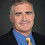## Wednesday, October 02, 2002

### Foundations of ComplexityLesson 3: Universal Turing Machines and Diagonalization

Previous Lesson | Next Lesson

A Universal Turing Machine is a machine so powerful that it can simulate any other Turing machine. Initially it seems amazing that such a machine can exist. But think about the microprocessor that sits on the computer you are now using. Every program that you use, your word processor, the spreadsheet, the browser, the mp3 player all use code that runs on this processor. This processor acts like a universal Turing machine. Another example, is an interpreter for a language like Java. Suppose we had a program written in C++. The Java interpreter can run code that lets it interprets C++ and thus run any C++ program. This works for any other language and thus a Java interpreter is also a universal Turing machine.

What we have done is to consider programs as data themselves. Fix a programming language. For a machine M let <M> be the binary encoding of the program describing M. Let LU be the set of pairs (<M>,x) such that machine M accepts input x. LU is a computably enumerable set as we can create a machine U that simulates M on input x. The machine U is a universal Turing machine.

We now show that there is a language that is not computably enumerable. Let LD be the set of <M> such that machine M does not accept <M>. Suppose LD is computably enumerable. There must be some machine N such that N(<M>) accepts if and only if <M> is in LD. We have two cases

1. N(<N>) accepts: <N> is in LD so by definition of LD, N does not accept <N>, a contradiction.
2. N(<N>) does not accept: <N> is not in LD so by definition of LD, N accepts <N>, a contradiction.
This kind of argument is called diagonalization. It is the main technique to show that problems cannot be computed.

Step back for a second. We have shown that the language LD cannot be computed by a computer. Any computer. Ever.

1.Already stuck on lesson 3... have not halted, accepted or rejected after 1 day. Continuing processing. Thanks for the lessons!

2.Hi, I'm studying some topics of computational complexity now, and there is one thing that seems hard to understand regarding relativization. I know it is not the right place to post this, but I think it is somehow related to diagonalization. So, here is my question:

Take for instance the class C=P^NP, and a language B. How are the oracle machines from C^B working?
- are they deterministic polytime machines that ask queries from NP^B?
- are they deterministic polytime machines that ask queries to both B and NP?

Thanks!

3.The rule is all machines must have access to oracle B. So in (P^NP)^B both the P and NP machines have access to B, think ((P^B)^(NP^B)). Now the P machine can access B through the NP machine so this is the same as P^(NP^B).

4.Thanks!